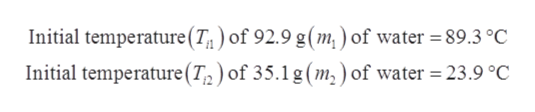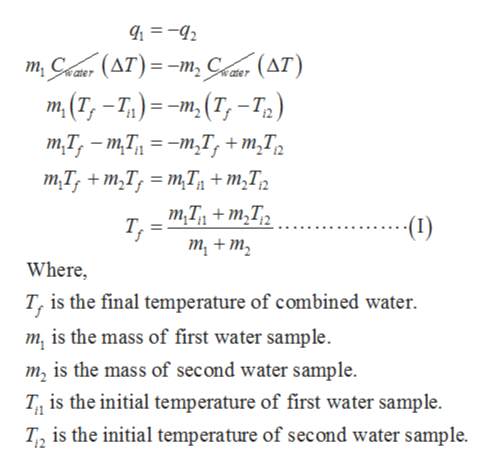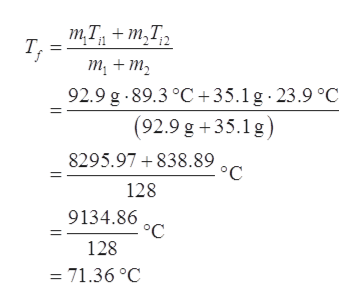# A student mixes 92.9 g of water at 89.3°C with 35.1 g of water at 23.9°C in an insulated flask. What is the final temperature of the combined water?

Question
2 views

A student mixes 92.9 g of water at 89.3°C with 35.1 g of water at 23.9°C in an insulated flask. What is the final temperature of the combined water?

check_circle

Step 1

Given data:help_outlineImage TranscriptioncloseInitial temperature (T ) of 92.9 g(m, ) of water = 89.3 °C Initial temperature (T2) of 35.1 g (m, ) of water 23.9 °C fullscreen
Step 2

Since heat transfers from 92.9 g of water to 35.1 g of water, the heat transfer (q) must be equal in magnitude but opposite sign. The final temperature of combined water is calculated as shown below.help_outlineImage Transcriptionclosem, Caar (AT)= -m Casr (AT) m(T,-T) -m(T, -T) тТ, - тТ, %3 -т,Т, +т,T, ater тT, + m,T, — тТ, + т,T, т,Т, + m,Tz Т, т, + т, (1)- Where, T, is the final temperature of combined water. m is the mass of first water sample m2 is the mass of second water sample T is the initial temperature of first water sample T2 is the initial temperature of second water sample. fullscreen
Step 3

Substitute the given data in equation (I) to calculate the ...help_outlineImage TranscriptioncloseтТ, + m,T, т, т, + т, 92.9 g.89.3°C+35.1 g 23.9 °C (92.9 g +35.1 g) 9°C 8295.97 838.89 128 9134.86 128 = 71.36 °C fullscreen

### Want to see the full answer?

See Solution

#### Want to see this answer and more?

Solutions are written by subject experts who are available 24/7. Questions are typically answered within 1 hour.*

See Solution
*Response times may vary by subject and question.
Tagged in

### Physical Chemistry